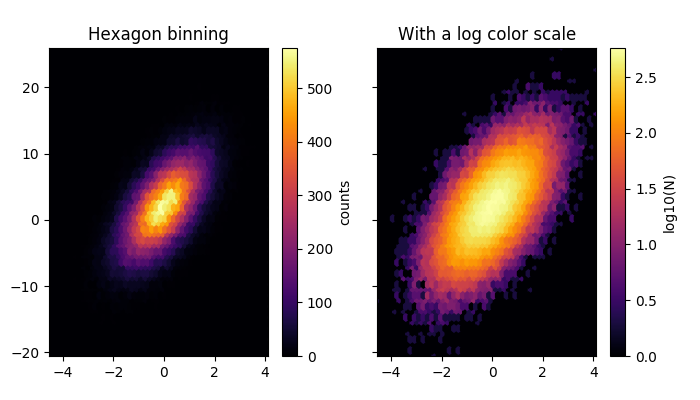# Hexbin Demo¶

Plotting hexbins with Matplotlib.

Hexbin is an axes method or pyplot function that is essentially a pcolor of a 2-D histogram with hexagonal cells. It can be much more informative than a scatter plot. In the first plot below, try substituting ‘scatter’ for ‘hexbin’.```import numpy as np
import matplotlib.pyplot as plt

# Fixing random state for reproducibility
np.random.seed(19680801)

n = 100000
x = np.random.standard_normal(n)
y = 2.0 + 3.0 * x + 4.0 * np.random.standard_normal(n)
xmin = x.min()
xmax = x.max()
ymin = y.min()
ymax = y.max()

fig, axs = plt.subplots(ncols=2, sharey=True, figsize=(7, 4))
ax = axs
hb = ax.hexbin(x, y, gridsize=50, cmap='inferno')
ax.axis([xmin, xmax, ymin, ymax])
ax.set_title("Hexagon binning")
cb = fig.colorbar(hb, ax=ax)
cb.set_label('counts')

ax = axs
hb = ax.hexbin(x, y, gridsize=50, bins='log', cmap='inferno')
ax.axis([xmin, xmax, ymin, ymax])
ax.set_title("With a log color scale")
cb = fig.colorbar(hb, ax=ax)
cb.set_label('log10(N)')

plt.show()
```

Keywords: matplotlib code example, codex, python plot, pyplot Gallery generated by Sphinx-Gallery